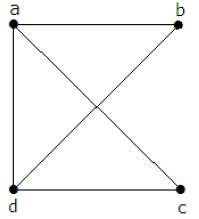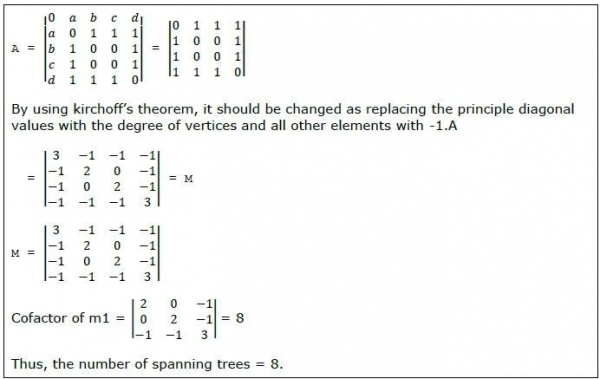# Kirchoff's Theorem

Kirchoff's theorem is useful in finding the number of spanning trees that can be formed from a connected graph.

## ExampleThe matrix 'A' be filled as, if there is an edge between two vertices, then it should be given as '1', else '0'.